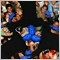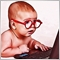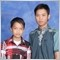# Incrementing Lot Size per AccountBalance() - page 220061

DeanDeV:

I simply would like something like this...

```if(AccountBalance()>=1000 && AccountBalance()<2000) Lots=0.1;
if(AccountBalance()>=2000 && AccountBalance()<3000) Lots=0.2;```

Any way to do this instead of typing them all out??

Lots = int(AccountBalance() / 1000) / 10.0;

However if you're not using a proper SL, then even 0.1 lots means your account is at risk (EURUSD 1.2345) \$12,345.00 (buy)840

DeanDeV:
Please excuse this if this is completely incorrect, however, is it possible to do something like this:
It is possible, I am sure.  The thing to remember, for a lot of the problems that people are trying to solve, there is usually more than one way to get the desired result.  The trick is finding out which of all of these possible solutions is the best one to use.  By best possible, in this case at least, I would consider how many lines it takes to solve the problem, because usually more code means more processing time.  And more processing time means the longer it takes to actually do anything as far as the purpose of the EA.41

You can do it like this....this EA for Example

```//+------------------------------------------------------------------+
//|Gap 30 minutes
//| EURUSD 1MN
//+------------------------------------------------------------------+
//---- input parameters
extern string mn="GAP-30-MN";
extern int MagicNumber=1992; //magic
extern int    min_gapsize = 5;
extern double LotFactor = 42; //lotsize factor
double lot;
//----
datetime order_time = 0;
//+------------------------------------------------------------------+
//| expert initialization function                                   |
//+------------------------------------------------------------------+
int init()
{
//----
return(0);
}
//+------------------------------------------------------------------+
//| expert deinitialization function                                 |
//+------------------------------------------------------------------+
int deinit()
{
//----
return(0);
}
//+------------------------------------------------------------------+
//| expert start function                                            |
//+------------------------------------------------------------------+
int start()
{
// Defining the variables to decide.
Print("order time", order_time);
double current_openprice = iOpen(Symbol(),PERIOD_M30, 0);
double previous_highprice = iHigh(Symbol(),PERIOD_M30, 1);
double previous_lowprice = iLow(Symbol(),PERIOD_M30, 1);
double point_gap = MarketInfo(Symbol(), MODE_POINT);
datetime current_time = iTime(Symbol(),PERIOD_M30, 0);
// catching the gap on sell upper gap
if(current_openprice > previous_highprice + (min_gapsize + spread_gap)*point_gap &&
current_time != order_time)
{
int ticket = OrderSend(Symbol(), OP_SELL, NR(Lot_Volume()), Bid, 0, 0,
"GAP-30-MN", MagicNumber, 0, Red);
order_time = iTime(Symbol(),PERIOD_M30, 0);
Print("I am inside (sell) :-)", order_time);
//----
if(ticket < 0)
{
Print("OrderSend failed with error #", GetLastError());
}
}
//catching the gap on buy down gap
if(current_openprice < previous_lowprice - (min_gapsize + spread_gap)*point_gap &&
current_time != order_time)
{
"GAP-30-MN", MagicNumber, 0, Green);
order_time = iTime(Symbol(),PERIOD_M30, 0);
Print("I am inside (buy) :-)", order_time);
if(ticket < 0)
{
Print("OrderSend failed with error #", GetLastError());
}
}
//----
return(0);
}

//+------------------------------------------------------------------+
//Calculates Lot Size based on balance and factor
//+------------------------------------------------------------------+
double NR(double thelot)
{
double maxlots=MarketInfo(Symbol(),MODE_MAXLOT),
minilot=MarketInfo(Symbol(),MODE_MINLOT),
lstep=MarketInfo(Symbol(),MODE_LOTSTEP);
double lots=lstep*NormalizeDouble(thelot/lstep,0);
lots=MathMax(MathMin(maxlots,lots),minilot);
return (lots);
}

//+------------------------------------------------------------------+
double Lot_Volume()
{

lot=AccountBalance() * 0.01 /LotFactor ;

return(lot);
}
//+------------------------------------------------------------------+```125

Can you help me,

I want to make lot size settings in accordance with the risk options

==============

double Lot_1 = ?? // Options from "Low_Risk or Med_Risk Or High_Risk

double SL_pips = 30;

==============

enum  Risk

{

Low_Risk,   Med_Risk,   High_Risk,

};

extern   Risk Risk_Options;

If i Choice Low_Risk, it mean i use Lot_1 = NormalizeDouble ((AccountBalance() * 5% / SL_Pips),2)

or

If i Choice Med_Risk, it mean i use Lot_1 = NormalizeDouble ((AccountBalance() * 10% / SL_Pips),2)

or

If i Choice High_Risk, it mean i use Lot_1 = NormalizeDouble ((AccountBalance() * 20% / SL_Pips),2)

====================================================================================